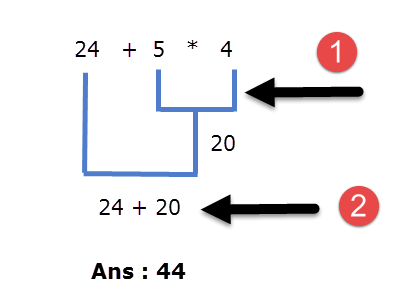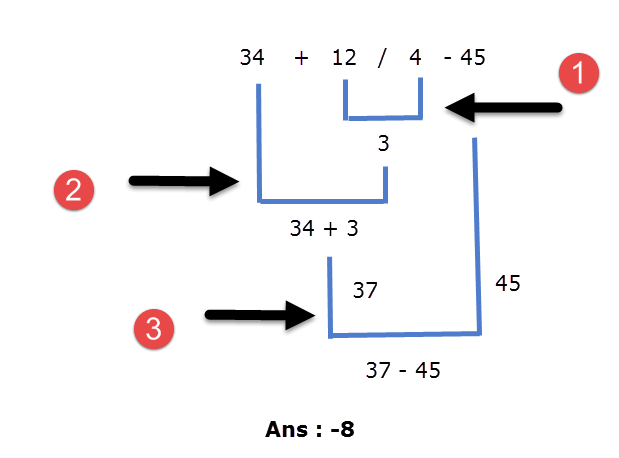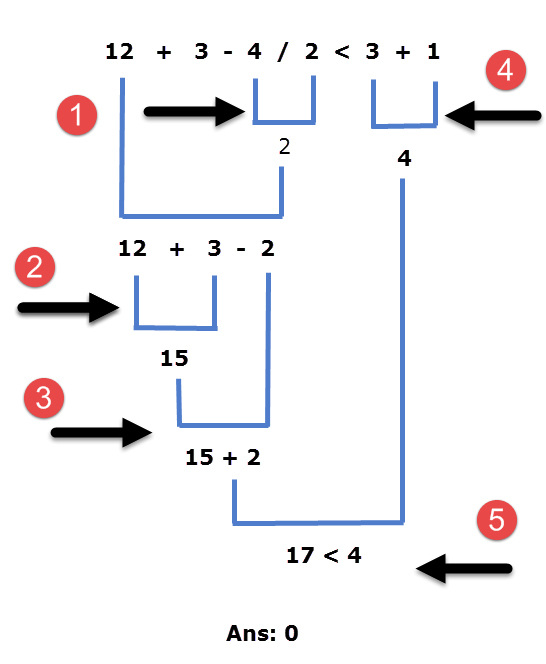# Operator Precedence and Associativity in C

Last updated on July 27, 2020

Operator precedence: It dictates the order of evaluation of operators in an expression.

Associativity: It defines the order in which operators of the same precedence are evaluated in an expression. Associativity can be either from left to right or right to left.

Consider the following example:

24 + 5 * 4


Here we have two operators + and *, Which operation do you think will be evaluated first, addition or multiplication? If the addition is applied first then answer will be 116 and if the multiplication is applied first answer will be 44. To answer such question we need to consult the operator precedence table.

In C, each operator has a fixed priority or precedence in relation to other operators. As a result, the operator with higher precedence is evaluated before the operator with lower precedence. Operators that appear in the same group have the same precedence. The following table lists operator precedence and associativity.Operators in the top have higher precedence and it decreases as we move towards the bottom.

From the precedence table, we can conclude that the * operator is above the + operator, so the * operator has higher precedence than the + operator, therefore in the expression 24 + 5 * 4, subexpression 5 * 4 will be evaluated first.Here are some more examples:

Example 1:

34 + 12/4 - 45Here the / operator has higher precedence hence 12/4 is evaluated first. The operators + and - have the same precedence because they are in the same group. So which one of them will be evaluated first? To solve this problem you need to consult the associativity of the operator. As you can see in the table, the operators + and - have the same precedence and associates from left to right therefore in our expression 34 + 12/4 - 45 after division, addition (+) will be performed before subtraction (-).

Example 2:

12 + 3 - 4 / 2 < 3 + 1Here the / operator has higher precedence hence 4/2 is evaluated first. The + and - operators have the same precedence and associates from left to right, therefore in our expression 12 + 3 - 4 / 2 < 3 + 1 after division, the + operator will be evaluated followed by the - operator. From the precedence table, you can see that precedence of the < operator is lower than that of /, + and -. Hence, it will be evaluated at last.

## Using Parentheses #

If you look at the precedence table you will find that the precedence of parentheses (()) operator is the highest. Consequently, just as we did in school, we can use parentheses to change the sequence of operations. Consider the following example:

3 + 4 * 2


Here, the * operator will be evaluated first followed by the + operator.

What if you want the addition to take place first followed by multiplication?

We can do this using parentheses, as follows:

(3 + 4) * 2


Whatever you have wrapped inside the parentheses will be evaluated first. As a result, in this expression the addition will take place first followed by multiplication.

You can also nest parentheses like this:

(2 + (3 + 2) ) * 10


In such cases expression inside the innermost parentheses is evaluated first, then the next innermost parentheses and so on.

We can also use parentheses to make complex expressions a little more readable. For example:

 1 2 age < 18 && height < 48 || age > 60 && height > 72 (age < 18 && height < 48) || (age > 60 && height > 72) // much better than the above 

Both expressions give the same result, but adding parentheses makes our intent much clear.

We haven't yet discussed relational and logical operators. So the above expression might not make perfect sense. Relational and Logical operators are discussed in detail in Relational Operators in C and Logical Operators in C, respectively. In the next chapter, we will learn about the if else statement in C.